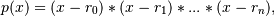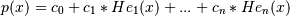# numpy.polynomial.hermite_e.hermefromroots¶

`numpy.polynomial.hermite_e.``hermefromroots`(roots)[source]

Generate a HermiteE series with given roots.

The function returns the coefficients of the polynomialin HermiteE form, where the r_n are the roots specified in roots. If a zero has multiplicity n, then it must appear in roots n times. For instance, if 2 is a root of multiplicity three and 3 is a root of multiplicity 2, then roots looks something like [2, 2, 2, 3, 3]. The roots can appear in any order.

If the returned coefficients are c, thenThe coefficient of the last term is not generally 1 for monic polynomials in HermiteE form.

Parameters: roots : array_like Sequence containing the roots. out : ndarray 1-D array of coefficients. If all roots are real then out is a real array, if some of the roots are complex, then out is complex even if all the coefficients in the result are real (see Examples below).

`polyfromroots`, `legfromroots`, `lagfromroots`, `hermfromroots`, `chebfromroots.`

Examples

```>>> from numpy.polynomial.hermite_e import hermefromroots, hermeval
>>> coef = hermefromroots((-1, 0, 1))
>>> hermeval((-1, 0, 1), coef)
array([ 0.,  0.,  0.])
>>> coef = hermefromroots((-1j, 1j))
>>> hermeval((-1j, 1j), coef)
array([ 0.+0.j,  0.+0.j])
```

#### Previous topic

numpy.polynomial.hermite_e.hermeroots

#### Next topic

numpy.polynomial.hermite_e.hermefit